American Journal of Civil Engineering
Volume 4, Issue 1, January 2016, Pages: 1-11

Experimental Testing and Analytical Modeling of Strip Footing in Reinforced Sandy Soil with Multi-Geogrid Layers Under Different Loading Conditions

Aram Mohammed Raheem1, *, Mohammed Abdulsalam Abdulkarem2

1Civil Engineering Department, University of Kirkuk, Kirkuk, Iraq

2Geotechnical Engineer, Ministry of Construction and Housing, Kirkuk, Iraq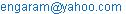(A. M.Raheem)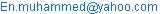(M. A. Abdulkarem)

Aram Mohammed Raheem, Mohammed Abdulsalam Abdulkarem. Experimental Testing and Analytical Modeling of Strip Footing in Reinforced Sandy Soil with Multi-Geogrid Layers Under Different Loading Conditions. American Journal of Civil Engineering. Vol. 4, No. 1, 2016, pp. 1-11. doi: 10.11648/j.ajce.20160401.11

Abstract: In this study, large-scale physical models with dimensions of (0.9m * 0.9m * 0.55m) have been designed and constructed to investigate the behavior of strip footing in reinforced sandy soil with multi-geogrid layers under inclined and eccentric loading conditions. The effect of several parameters such as geogrid layers (N), soil relative density (RD), depth of the topmost geogrid layer (U/B), load inclination angle (a) and load eccentricity ratio (e/B) on the bearing capacity ratio (BCR) of reinforced soil have been investigated through 120 experimental tests. As the number of the geogrid layers increased from 0 to 4, the BCR increased by 255% for 15o load inclination angle and by 470% for 0.05 load eccentricity ratio in 60% RD. When the RD of the soil increased from 60% to 80%, the average decreases in horizontal displacement and footing tilting angle were about 35% and 21% respectively. Hyperbolic analytical model was used to predict the relationships of most of the studied parameters. However, p-q analytical model was suggested to model the relationship between the BCR versus U/B. Both suggested models (hyperbolic and p-q) were in a very good agreement with the experimental results.

Keywords: Strip Footing, Experimental Study, Sandy Soil, Geogrid, Analytical Models, Different Loading Condition

Contents

1. Introduction

Several numerical attempts have been done to study the stability of reinforced soil mass as a homogenous anisotropic material were analyzed through rigid plastic FEM [19, 20]. Furthermore, a numerical study was used through FLAC software to study the effect of geosynthetic reinforcement arrangement on two square footings on sandy soil [21-23]. Rarely, analytical models were used to investigate the behavior of reinforced sandy soil with geogrid layers.

2. Objectives

The overall objective of this study was to model the behavior of strip footing rested on reinforced sandy soil with different layers of geogrid under the influence of inclined and eccentric loading conditions. The specific objectives were as follows:

1. Perform large-scale laboratory testing of strip footing on reinforced sandy soil with geogrid layers.

2. Study the effects of geogrid layers (N), soil relative density (RD), depth of the topmost geogrid layer (U/B), load inclination angle (a) and load eccentricity ratio (e/B) on the bearing capacity ratio (BCR) of reinforced soil.

3.  Investigate the validation of analytical models to predict BCR, horizontal displacement and tilting angle of strip footing over reinforced sandy soil under different loading conditions.

3. Materials and Methods

3.1. Laboratory Model Tests

3.1.1. Model Test Tank

The soil layers were prepared in a steel box with 0.9m × 0.9m and 0.55m dimensions made with a plate thickness of 6 mm supported by four steel channels as shown in Fig. 1. The inner faces of the steel box were painted to minimize the slide friction between the soil and steel box that may develop during experimental testing. Several lines were marked to identify carefully the required thickness of the soil layers and the location of the geogrid.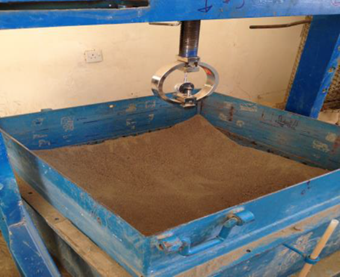Fig. 1. Laboratory testing box.

3.1.2. Footing

A strip steel channel of 80 mm in the plan with a thickness of 4 mm was used to represent the tested footing as shown in Fig. 2. The transferred load to the footing was measured with a proving ring of 5 kN capacity. Both horizontal and vertical displacements were measured using three dial gauges (0.01 mm/ division). The footing size was made based on the size of the steel model tank and the zone of the influence. Detailed testing instrumentations including dial gauges, proving ring and strip footing are shown in Fig. 3.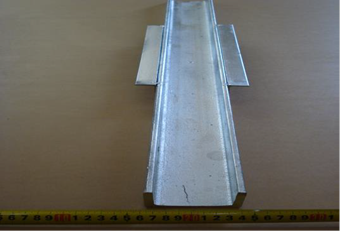Fig. 2. Strip footing represented by steel channel.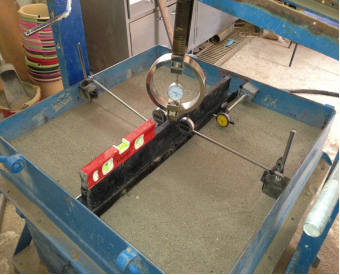Fig. 3. Detailed testing instrumentations.

3.2. Test Material

3.2.1. Sand Properties

A poorly graded sand passing sieve No.4 was used in this study. The sand was washed with running water to remove the dust as much as possible. Testing has been performed with dense and medium dense sand corresponding to approximately (16.9) kN/m3 and (17.5) kN/m3 consistent with relative densities of (60) % and (80) % respectively. The maximum and minimum dry unit weights of the sand were determined according to the ASTM (D4253-00) and ASTM (D4254-00), respectively.

The results have shown maximum and minimum dry unit weight of sand as 18 kN/m3 and 15.6 kN/m3 respectively. The specific gravity of the sand was 2.59 and the test has been done based on the ASTM D-854. The grain size distribution analysis of the sand was performed according to the ASTM D-421 and it can be shown in Fig. 4. The sand was classified according to the unified soil classification system as poorly graded sand with coefficient of uniformity (Cu) = 3.0 and coefficient of curvature (CC) = 1.0.

3.2.2. Geogrid

One type of commercially available geogrid type was used TriAx® TX140 Geogrid manufactured from a punched polypropylene sheet, which was oriented in three significantly equilateral directions so that the subsequent ribs shall have a high degree of molecular orientation. The properties influencing the performance of a mechanically stabilized layer are summarized in Table 1.

Table 1. Engineering properties of Tenax TT Samp geogrid.

 Index Properties Longitudinal Diagonal Transverse Rib pitch, mm(in) 40(1.6) 40(1.6) - Mid-rib depth mm(in) - 1.2(0.05) 1.2(0.05) Mid-rib width mm(in) - 1.1(0.04) 1.1(0.04)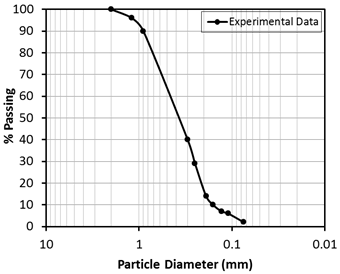Fig. 4. Particle size distribution of tested sand.

4. Testing Program

Detailed testing program has been established to reach the aim of the study of the effect of load applied on strip footings on reinforced sand. The parameters were loads eccentricity (e/B), loads inclination (α), number of geogrids layers (N), depth of topmost layer (U/B) and relative density (RD) which varied from a test to another. For all the tests, the footing was rested on the surface of the sandy soil and the distance between consecutive layers kept constant with a value of 0.05 m. The maximum number of geogrid layers that used in this study was four. The embedment length for the geogrid layers was 0.8 m. A schematic diagram for the strip footing in the sandy soil is shown in Fig. 5.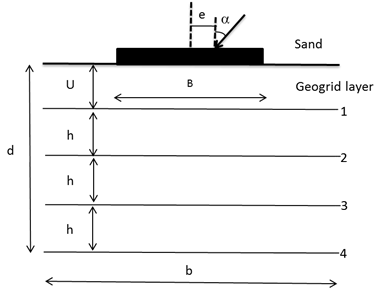Fig. 5. Schematic diagram for tested strip footing on reinforced sandy soil.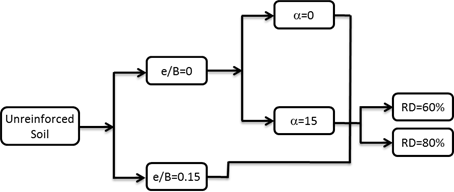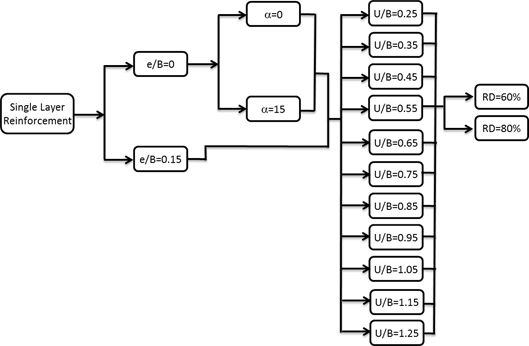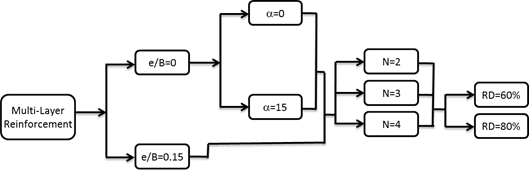Fig. 6. Flow chart for the testing program.

Fig. 6 shows the flow chart of the testing program. The flow chart was divided into three parts, the first part included the experimental work of unreinforced soil where it can be used as a reference to compare the improvement of using a geogrid as a reinforcement. In addition, it was used to study the effect of changing the load inclination and eccentricity on the bearing capacity of unreinforced sand for two studied relative densities. The second part focused on a single layer of reinforcement where these tests were used to study and locate the optimum depth of the topmost layer of geogrid (U/B). The third part, which was the main emphasis of this study, showed the effect of the multi-reinforcement layer on the bearing capacity including the effect of the load inclination and eccentricity on the optimum number of the reinforcement layer. The term bearing capacity ratio (BCR) is used to express the combined effect of soil reinforcement with load inclined and eccentricity on bearing capacity and it can be shown as follows: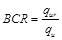(1)

where qur is the ultimate bearing capacity of inclined and eccentrically load strip footing on reinforced sand while qu is the ultimate baring capacity of strip footing on unreinforced sand.

Meyerhof  suggested an empirical relation to compute the ultimate bearing capacity of footings subjected to eccentric-inclined loads:(2)

where C,g are the soil cohesion and density respectively. Nc, Nq, and Ng are bearing capacity factors. Sc, Sq, and Sg are shape factors. Dc, Dq, and Dg are depth factors. Ic, Iq, and Ig are inclined load factors. B is the footing width. D is footing embedment depth.

5. Analytical Models and Prediction

Based on the experimental expectation results, the following analytical models can be used:

5.1. Hyperbolic Model

For reinforced soil, the bearing capacity ratio (BCR) versus number of geogrid layers (N) is expected to increase to a certain level where beyond that point no increase in BCR could be anticipated even if N increased. Such kind of relationships has been noticed in several engineering and environmental applications and modeled using hyperbolic model. In early stages of developing hyperbolic model, it was used to predict the amount of phenol leached from a solidified cement matrix . Furthermore, hyperbolic model was used to represent the relationship between the changes in grouted sand properties with curing time . Vipulanandan et al.  proposed hyperbolic relationship to characterize the variation of in-situ vertical stress and logarithmic undrained shear strength of the soft marine and deltaic clays. Hyperbolic relationship can be used to correlate the compressive strength variation with curing time for cemented sand . Hyperbolic model were used for several other relationships such as fluid loss versus time in high-pressure high-temperature condition , shear strength versus solid content of ultra-soft soil , and changes in electrical resistivity versus salt content of bentonite drilling mud . The hyperbolic model formulation is as follows: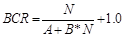(3)

where A & B are model parameters while BCR and N are bearing capacity ratio and number of geogrid layers respectively.

5.2. P-q Model

It is expected that the bearing capacity ratio (BCR) will increase with increasing the depth of the topmost layer of geogrid (U/B) to the optimum value then it will start to decrease with increasing U/B value. Such nature of relationship can be modeled using p-q model. This model was first proposed by Mebarkia and Vipulanandan  to predict the stress-strain behavior of glass-fiber-reinforced polymer concrete. The original p-q model formulation is as follows: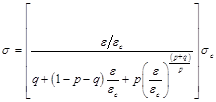(4)

where s = compressive stress, sc, ec = compressive strength and its corresponding strain, p, q = model parameters.

This model has been adopted in this study to model the relationship between BCR and U/B as follows:(5)

where (BCR)c, (U/B)c = maximum (BCR) and its corresponding (U/B).

5.3. Comparison of Model Prediction

In order to determine the accuracy of the model predictions, both coefficient of determination (R2) and the root mean square error (RMSE) in curve fitting as defined in Eqs. (6) and (7) were quantified.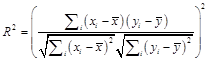(6)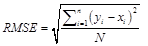(7)

where yi is the actual value; xi is the calculated value from the model;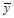is the mean of actual values;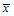is the mean of calculated values and N is the number of data points.

6. Results and Analysis

6.1. BCR Versus N

In this section, the BCR versus N for load inclination angle (a) varied from 5° to 15° of two different RD (60% and 80%) can be identified clearly in Fig. 7 (a to d). In Fig. 7 (a) and 7 (b), the relationship between the BCR and N for zero load inclination for strip footing in 60% and 80% RD are shown respectively. As the RD of the soil increased from 60% to 80%, the average increase in BCR was about 17%. The hyperbolic model predicted the experimental data preciously with R2 and RMSE of 0.99, 0.078, 0.98 and 0.131 for 60% and 80% RD respectively. At higher load inclination angle (a=15), the BCR decreased as the RD of the soil increased and the average decrease was about 43% as shown in Fig. 7 (c) and 7 (d). The R2 and RMSE of the hyperbolic model were 0.94, 0.242, 0.99 and 0.09 for 60% and 80% RD respectively. The overall behavior implied that having higher number of geogrid layers increased the BCR of sandy soil while the load inclination effect was more at higher RD. As the geogrid layer increased from 0 to 4, the BCR increased by 210%, and 250% for zero load inclination and by 255%, and 100% for 15o load inclination for RD of 60% and 80% respectively. Hyperbolic model parameters that used to predict the relationship between BCR and N for different load inclination (Fig. 7) can be summarized in Table 2.

Table 2. Model parameters of hyperbolic model (Fig. 7).

 a RD(%) A B R2 RMSE 0 60 0.3 0.4 0.99 0.078 0 80 0.5 0.3 0.98 0.131 15 60 0.85 0.18 0.94 0.242 15 80 1 0.9 0.99 0.090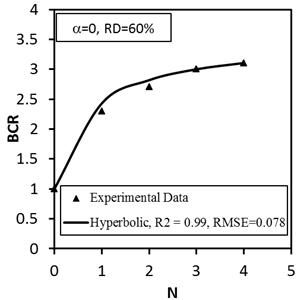(a)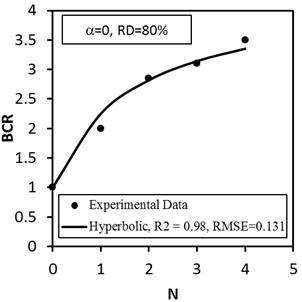(b)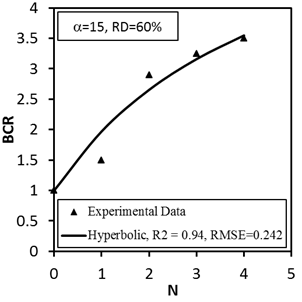(c)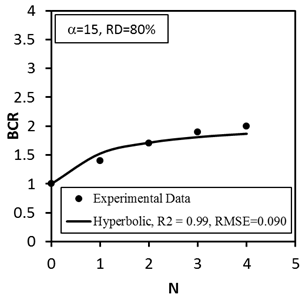(d)

Fig. 7. Modeling of BCR versus N relationship of strip footing rested on sandy soil.

(a) a=0, RD=60%, (b) a=0, RD=80%, (c) a=15, RD=60%, and (d) a=15, RD=80%.

In this section, the BCR versus N for load eccentricity ratio (e/B) varied from 0 to 0.15 of two different RD (60% and 80%) can be displayed clearly in Fig. 8 (a to d). In Fig. 8 (a) and 8 (b), the relationship between the BCR and N for zero load eccentricity ratio of strip footing in 60% and 80% RD are shown respectively. As the RD of the soil increased from 60% to 80%, the average increase in BCR was about 23%. The hyperbolic model predicted the experimental data preciously with R2 and RMSE of 0.97, 0.099, 0.98 and 0.135 for 60% and 80% RD respectively. At higher load eccentricity (e/B=0.15), the BCR decreased as the RD of the soil increased and the average decrease was about 45% as shown in Fig. 8 (c) and 8 (d). The R2 and RMSE of the hyperbolic model were 0.96, 0.335, 0.98 and 0.250 for 60% and 80% RD respectively. As the geogrid layer increased from 0 to 4, the BCR increased by 180%, and 260% for zero load eccentricity and by 470%, and 185% for 0.05 load eccentricity for RD of 60% and 80% respectively. Hyperbolic model parameters that used to predict the relationship between BCR and N for different load eccentricity (Fig. 8) can be identified in Table 3.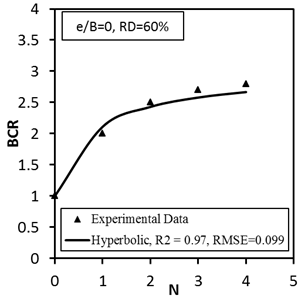(a)(b)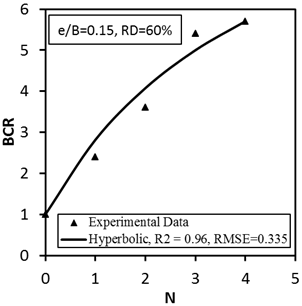(c)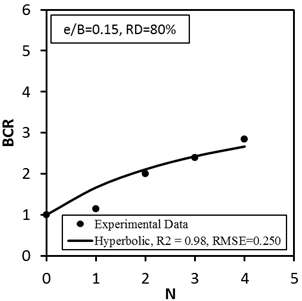(d)

Fig. 8. Modeling of BCR versus N relationship of strip footing rested on sandy soil.

(a) e/B=0, RD=60%, (b) e/B=0, RD=80%, (c) e/B=0.15, RD=60%, and (d) e/B=0.15, RD=80%.

Table 3. Model parameters of hyperbolic model (Fig. 8).

 e/B RD(%) A B R2 RMSE 0 60 0.4 0.5 0.97 0.099 0 80 1 0.15 0.98 0.135 0.15 60 0.45 0.1 0.96 0.335 0.15 80 1.2 0.3 0.98 0.250

6.2. Horizontal Displacement Versus N

In this section, the horizontal displacement of the strip footing versus N for load inclination (a) varied from 0o to 15o of two different RD (60% and 80%) can be presented obviously in Fig. 9 (a to d). In Fig. 9 (a) and 9 (b), the relationship between the horizontal displacement and N for 5o load inclination of strip footing in 60% and 80% RD are shown respectively. As the RD of the soil increased from 60% to 80%, the average decrease in the horizontal displacement was about 35%. The hyperbolic model predicted the experimental data preciously with R2 and RMSE of 0.99, 0.094 mm, 0.99 and 0.011 mm for 60% and 80% RD respectively. At higher load inclination (a=15o), the horizontal displacement decreased as the RD of the soil increased and the average decrease was about 45% as shown in Fig. 9 (c) and 9 (d). The R2 and RMSE of the hyperbolic model were 0.96, 0.335 mm, 0.98 and 0.250 mm for 60% and 80% RD respectively. The overall behavior suggested that the load eccentricity effect was further at higher RD. As the geogrid layer increased from 0 to 4, the horizontal displacement of the strip footing decreased by 62%, 14% for 5o load inclination and by 54%, 69% for 15o load inclination for RD of 60% and 80% respectively. Hyperbolic model parameters that used to predict the relationship between the horizontal displacement of the strip footing and N for different load inclination (Fig. 9) can be shown in Table 4.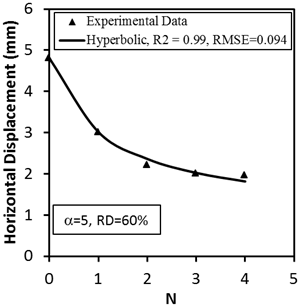(a)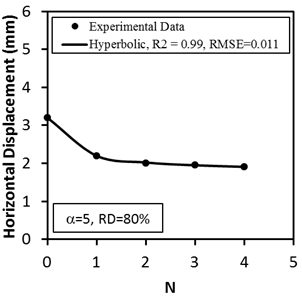(b)(c)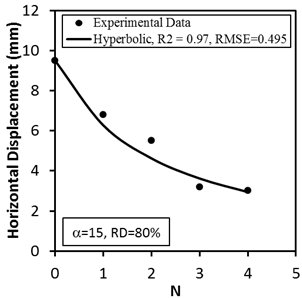(d)

Fig. 9. Modeling of horizontal displacement versus N relationship of strip footing rested on sandy soil (a) α=5, RD=60%, (b) α=5, RD=80%, (c) α=15, RD=60%, and (d) α=15, RD=80%.

Table 4. Model parameters of hyperbolic model (Fig. 9).

 a RD(%) A B R2 RMSE (mm) 5 60 -0.3 -0.26 0.99 0.094 5 80 -0.3 -0.7 0.99 0.011 15 60 -0.22 -0.12 0.99 0.257 15 80 -0.21 -0.1 0.97 0.495

6.3. Tilting Angle Versus N

In this section, the strip footing tilting angle versus N for load eccentricity (e/B) varied from 0.05 to 0.15 of two different RD (60% and 80%) can be demonstrated obviously in Fig. 10 (a to d). In Fig. 10 (a) and 10 (b), the relationship between the strip footing tilting angle and N for 0.05 load eccentricity of strip footing in 60% and 80% RD are shown respectively. As the RD of the soil increased from 60% to 80%, the average decrease in the strip footing tilting angle was about 21%. The hyperbolic model predicted the experimental data preciously with R2 and RMSE of 0.95, 0.081o, 0.98 and 0.116o for 60% and 80% RD respectively. At higher load eccentricity (e/B=0.15), the strip footing tilting decreased as the RD of the soil increased and the average decrease was about 17% as shown in Fig. 10 (c) and 10 (d). The R2 and RMSE of the hyperbolic model were 0.98, 0.018o, 0.99 and 0.044o for 60% and 80% RD respectively. The overall behavior implied that having higher RD decreases the strip footing tilting angle regardless to the number of the geogrid layers. As the geogrid layer increased from 0 to 4, the strip footing titling angle increased by 90%, 275% for 0.05 load eccentricity and by 12%, 16% for 0.15 load eccentricity for RD of 60% and 80% respectively. Hyperbolic model parameters that used to predict the relationship between the footing tilting angle and N for different load eccentricity (Fig. 10) can be shown in Table 5.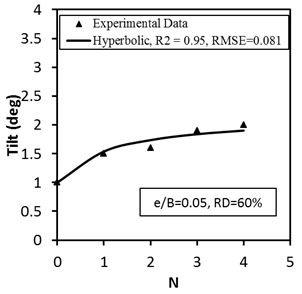(a)(b)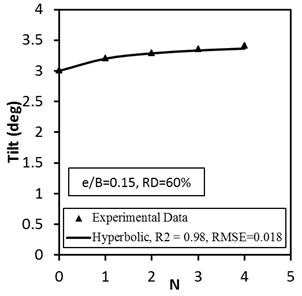(c)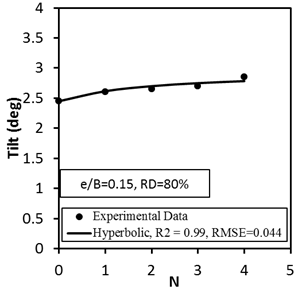(d)

Fig. 10. Modeling of footing tilting versus N relationship of strip footing rested on sandy soil (a) e/B=0.05, RD=60%, (b) e/B=0.05, RD=80%, (c) e/B=0.15, RD=60%, and (d) e/B=0.15, RD=80%.

Table 5. Model parameters of hyperbolic model (Fig. 10).

 e/B RD(%) A B R2 RMSE (o) 0.05 60 1 0.86 0.95 0.081 0.05 80 2.5 0.5 0.98 0.116 0.15 60 3 2 0.98 0.018 0.15 80 4 2 0.99 0.044

6.4. BCR Versus (U/B)

In this section, the BCR versus U/B for load inclination angle (a) varied from 0o to 15o of two different RD (60% and 80%) can be presented clearly in Fig. 11 (a to d). In Fig. 11 (a) and 11 (b), the relationship between BCR and U/B for 0o load inclination angle of strip footing in 60% and 80% RD are shown respectively. As the RD of the soil increased from  60% to 80%, the maximum decrease in BCR was about 14%. The p-q model predicted the experimental data preciously with R2 and RMSE of 0.97, 0.0238, 0.91 and 0.0219 for 60% and 80% RD respectively. At higher load inclination angle (a=15o), the BCR decreased as the RD of the soil increased and the maximum decrease was about 22% as shown in Fig. 11 (c) and 11 (d). The R2 and RMSE of the p-q model were 0.96, 0.0435, 0.96 and 0.0199 for 60% and 80% RD respectively. The overall behavior suggested that the load inclination angle effect was less at higher RD. P-q model parameters that used to predict the relationship between BCR and U/B for different load inclination (Fig. 11) can be shown in Table 6.

Table 6. Model parameters of p-q model (Fig. 11).

 a RD(%) p q R2 RMSE 0 60 1.1 1.15 0.97 0.0238 0 80 8 1.8 0.91 0.0219 15 60 0.9 1.2 0.96 0.0435 15 80 2 1.5 0.96 0.0199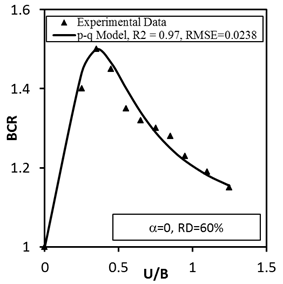(a)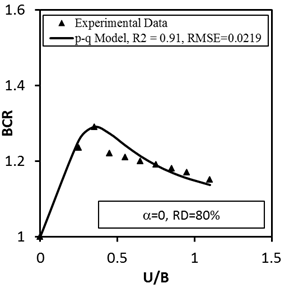(b)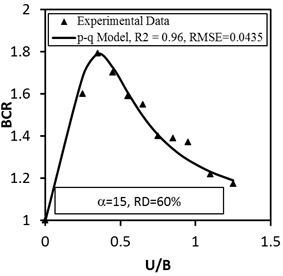(c)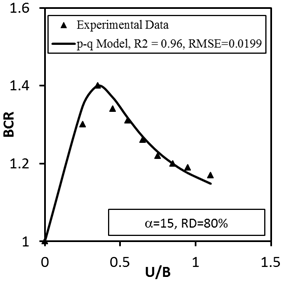(d)

Fig. 11. Modeling of BCR versus U/B relationship of strip footing rested on sandy soil (a) a=0, RD=60%, (b) a=0, RD=80%, (c) a=15, RD=60%, and (d) a=15, RD=80%.

7. Conclusions

Based on the main results of the study, the following conclusions can be advanced:

1. Using geogrid for soil reinforcement has a significant impact in increasing the ultimate bearing capacity of the cohesionless soil.

2. Increasing the number of geogrid layers (N) increases the ultimate bearing capacity ratio notably and this increase reaches 255% for 15o load inclination angle and 470% for 0.05 load eccentricity ratio in 60% RD.

3. Having higher numbers of geogrid layers (N) decreased both the horizontal displacement and the footing tilt. When the RD of the soil increased from 60% to 80%, the average decreases in the horizontal displacement and footing tilting were about 35% and 21% respectively.

4. The optimum value for U/B was about 0.5 and the BCR at this value (optimum) decreased as the RD increased. When the RD of the soil increased from 60% to 80%, the BCR at optimum U/B (0.5) decreased by 14% and 22% for 0o and 15o load inclination angle respectively.

5. The main factors affecting the ultimate bearing capacity of a strip footing under inclined and eccentric load on geogrid-reinforced sand can be addressed as follows:

a  The load inclination angle (α)

Increasing (α) decreased the ultimate bearing capacity.

Increasing (α) increased the horizontal displacement of the footing.

b  The load eccentricity ratio (e/B)

Increasing (e) decreased the ultimate bearing capacity.

Increasing (e) increased the tilting of the footing.

c  The Relative density (RD)

Increasing (RD) increased the ultimate bearing capacity.

Increasing (RD) decreased the horizontal displacement of the footing.

Most of the studied relationships such as BCR versus N for different load inclination angle, BCR versus N for different load eccentricity ratio, footing horizontal displacement versus N and footing tilting versus N were modeled using hyperbolic model. However, p-q model was used to model the relationship between the BCR versus U/B. Both suggested models (hyperbolic and p-q) were in a very good agreement with the experimental results.

Acknowledgment

The civil engineering department at the University of Tikrit in Iraq supported the experimental part of this study. This support is gratefully acknowledged.

References

1. El Sawwaf M. (2009). "Experimental and Numerical Study of Eccentrically Loaded Strip Footings Resting on Reinforced Sand," Journal of Geotechnical and Geoenvironmental Engineering ASCE, 135(10), 1509-1517, DOI: 10.1061/ASCEGT.1943-5606.0000093.
2. LU Liang, Wang Zong-Jian and K. Arai (2014). "Numerical and Experimental Analyses for Bearing Capacity of Rigid Strip Footing Subjected to Eccentric Load," J. Cent. South Univ., 21, 3983−3992, DOI: 10.1007/s11771-014-2386-5.
3. Dewaikar D.M., Guptha K.G. and Chore H.S. (2011). "Behavior of Eccentrically Loaded Model Square Footing on Reinforced Soil: An Experimental Investigation," Proceedings of Indian Geotechnical Conference, December 15-17, Kochi (Paper No. D-380).
4. Meyerhof G. G. (1953). "The Bearing Capacity of Footings under Eccentric and Inclined Loads," Proc., 3rd Int. Conf. on Soil Mech. and Found. Engrg., 1, 440–445.
5. Mahiyar H. and Patel A. N. (2000). "Analysis of Angle Shaped Footing under Eccentric Loading," J. Geotech. Geoenviron. Eng., 126(12), 1151–1156, DOI: 10.1061/(ASCE)1090-0241(2000)126:12(1151).
6. Zhang M.X., Qiu C.C., Javadi A. A. and Zhang S. L. (2014)." Model Tests on Reinforced Sloped Embankment with Denti-Strip Inclusions under Monotonic Loading," KSCE Journal of Civil Engineering, 18(5), 1342-1350, DOI: 10.1007/s12205-014-0222-y.
7. Guido V. A., Chang D. K., and Sweeney M. A. (1986). "Comparison of Geogrid and Geotextile Reinforced Earth Slabs," Can. Geotech. J., 23(4), 435–440., DOI: 10.1139/t86-073.
8. Huang C.C. and Tatsuoka K. (1990). "Bearing capacity of reinforced horizontal sandy ground," Geotextile and Geomembranes, 9(1), 51-82.
9. Yoo W., Kim B. and Cho W. (2015). "Model Test Study on the Behavior of Geotextile-Encased Sand Pile in Soft Clay Ground," KSCE Journal of Civil Engineering, 19(3), 592-601, DOI: 10.1007/s12205-012-0473-4.
10. Chakraborty D. and Kumar J. (2014). "Bearing Capacity of Strip Foundations in Reinforced Soils," International Journal of Geomechanics, 14(1), February 1, 45-58. DOI: 10.1061/(ASCE)GM.1943- 5622.0000275.
11. Yetimoglu T., Jonathan T. H. Wu and Ahmet S. (1994). "Bearing Capacity of Rectangular Footings on Geogrid-Reinforced Sand," Journal of Geotechnical Engineering, 120(12), 2083-2099, DOI: 10.1061/(ASCE)0733-9410(1994)120:12(2083).
12. Binquet J. and Lee K. L. (1975). "Bearing Capacity Tests on Reinforced Earth Slabs," J. Geotech. Engrg. Div., 101(12), 1241–1255.
13. Fragaszy R. and Lawton E. (1984). "Bearing Capacity of Reinforced Sand Subgrades," J. Geotech. Engrg., 1500–1507, DOI: 10.1061/(ASCE)0733-9410(1984)110:10(1500).
14. Khing K., Das B. M., Puri V. K., Cook E. E., and Yen S. C. (1993). "The Bearing Capacity of A Strip Foundation on Geogrid-Reinforced Sand," Geotext. Geomembr., 12(4), 351–361.
15. Omar M. T., Das B. M., Puri V. K., and Yen S. C. (1993). "Ultimate Bearing Capacity of Shallow Foundations on Sand With Geogrid Reinforcement," Can. Geotech. J., 30(3), 545–549, DOI: 10.1139/t93-046.
16. Shin E.C., Das B. M., Puri V. K., Yen S.-C. and Cook E. E. (1993). "Bearing Capacity of Strip Foundation on Geogrid-Reinforced Clay," J. ASTM Geotech Test., 16(4), 534–541, Paper ID GTJ10293J.
17. Das B. M., Shin E. C. and Omar M. T. (1994). "The Bearing Capacity of Surface Strip Foundation on Geogrid-Reinforced Sand and Clay—A Comparative Study," Geotech. Geol. Eng., 12(1), 1–14.
18. Das B. M. and Omar M. T. (1994). "The Effects of Foundation Width on Model Tests For The Bearing Capacity of Sand With Geogrid Reinforcement," Geotech. Geol. Eng., 12(2), 133–141.
19. Asaoka A., Kodaka T. and Pokhaerl G. (1994). "Stability Analysis of Reinforced Soil Structures Using Rigid Plastic Finite Element Method," Soils Found., 34(1), 107–118.
20. Ochiai H., Otani J., Hayashic S. and Hirai, T. (1996). "The Pull-Out Resistance of Geogrids in Reinforced Soil," Geotextiles and Geomembranes, 14(1), 19-42, DOI: S0266-1144(96)00027- I.
21. Ghazavi M and Lavasan A.A. (2008). "Interference Effect of Shallow Foundations Constructed on Sand Reinforced With Geosynthetics," Geotext Geomembr, 26, 404–415, DOI: 10.1016/j.geotexmem.2008.02.003.
22. Reza N. and Ebrahim M. (2014). "Bearing Capacity of Two Close Strip Footings on Soft Clay Reinforced With Geotextile," Arab J Geosci, 7, 623–639, DOI: 10.1007/s12517-012-0771-7.
23. Won M.S., Ling H.I. and Kim Y.S. (2004). "A Study of the Deformation of Flexible Pipes Buried Under Model Reinforced Sand," KSCE Journal of Civil Engineering, 8(4), 377-385, DOI: 10.1007/BF02829161.
24. Vipulanandan C. and Kirshnan S. (1993). "XRD Analysis and Leachability of Solidified Phenol–Cement Mixtures," Cem. Concr.Res., 23,792–802, DOI: 10.1016/0008-8846(93)90033-6.
25. Ata A. and Vipulanandan C. (1998). "Cohesive and Adhesive Properties of Silicate Grout on Grouted – Sand Behavior," J. Geotech. Geoenviron. Eng., 124(1), 38–44, DOI: 10.1061/(ASCE)1090-0241(1998)124:1(38)).
26. Vipulanandan C.,AhossinY.J. and Bilgin O. (2007). "Geotechnical Properties of Marine and Deltaic Soft Clays," GSP173 Adv. Meas. Model. Soil Behav., 1–13, DOI: 10.1061/40917(236)5.
27. Usluogullari O.,VipulanandanC. (2011). "Stress–Strain Behavior and California Bearing Ratio of Artificially Cemented Sand."J. Test. Eval., 39(4),1–9, Paper ID JTE103165.
28. Vipulanandan C., Raheem A. M., Basirat B., Mohammed A. and Richardson D. (2014)." New Kinetic Model to Characterize the Filter Cake Formation and Fluid Loss in HPHT Process", OTC, 25100-MS, Houston, TX, 5-8 May, 1-17, DOI: 10.4043/25100-MS.
29. Raheem A.M and Vipulanandan C. (2014). "Effect of Salt Contamination on the Bentonite Drilling Mud Shear Strength and Electrical Resistivity," THC Proceedings Conference & Exhibition, Houston, TX, USA.
30. Vipulanandan C. and Raheem A.M. (2015). "Rapid Detection of Salt Contamination in Bentonite Drilling Mud in Deep Oil Well Applications," AADE National Technical Conference and Exhibition, San Antonio, Texas, April 8-9, 15-NTCE-30, pp. 1-7.
31. Mebarkia S. and Vipulanandan C. (1992). "Compressive Behavior of Glass-Fiber-Reinforced Polymer Concrete," J Mater Civ Eng, 4(1), 91–105, DOI: 10.1061/(ASCE)0899-1561(1992)4:1(91).

 Contents 1. 2. 3. 3.1. 3.2. 4. 5. 5.1. 5.2. 5.3. 6. 6.1. 6.2. 6.3. 6.4. 7.
Article ToolsAbstractPDF(4104K)